Energy and Power

# Energy and Power - Science Class 9

 Table of contentsEnergyAre Various Energy Forms Interconvertible?Power (Rate of Doing Work)Commercial Unit of EnergyKilowatt-hour (kWh)Competitive Window## Energy

• When a car runs, the engine of the car generates a force that displaces the car. In other words, work is done by the car. This work is done on the expense of fuel. Fuel provides the energy needed to run the car. Had the petrol tank been empty, car could not be run. The conclusion is that, if there is no source of energy, no work will be done.
• Example: Suppose a lift takes some persons from ground floor to second floor. Then the lift performs work. If you enquire, you will find that the lift is operated by an electrical motor. Thus, electrical energy does the work. If there is no electricity the lift will not operate. Again if there is no source of energy, no work will be done.
• The above statement is not just true for the above example but is true for all processes. Therefore, energy is defined as the capacity to do work.
• More is the energy in a body, more is the work the body can do.
• Energy is a scalar quantity.
• The S.I. unit of energy is joule (J).
• Bigger units is 1 kJ = 1000 J, 1 MJ = 106 J
• The C.G.S. unit of energy is erg.

Memorise: Energy is the ability to do work. More the energy, more the work that can be performed and vice-versa.

### Forms of Energy

Nature has been very kind to us in providing us energy in various forms. These forms of energy are as follows.

1. Solar energy. The energy radiated by the Sun is called solar energy. Plants collect and store this energy to make food through photosynthesis.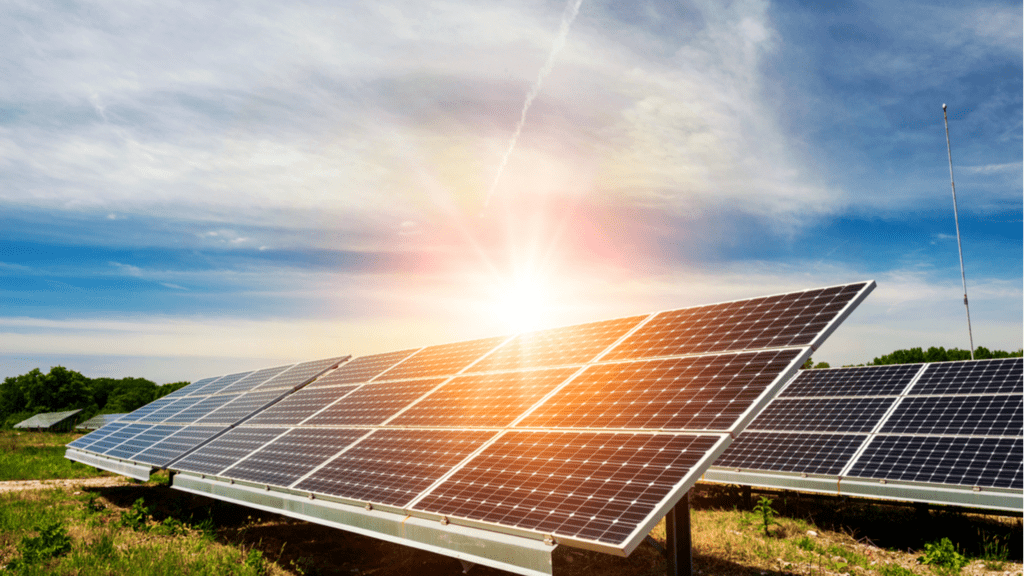Solar Energy

2. Heat energy. It is the energy released when coal, oil, gas or wood burn and it produces in us the sensation of warmth.

3. Light energy. It is the form of energy which produces in us the sensation of light. Sun is the natural source of light.

4. Chemical energy. It is the energy possessed by fossil fuels (coal; petroleum and natural gas) and is also called the fuel energy. The food that we eat has chemical energy stored in it.

5. Hydro energy. The energy possessed by water flowing in rivers and streams is called hydro energy. This energy is used to generate electricity in hydroelectric power plants.

6. Wind energy. The energy possessed by moving air is called wind energy.

7. Ocean thermal energy (OTE). Solar energy stored in the oceans in the form of heat is called ocean thermal energy.

8. Geothermal energy. It is the heat energy of the Earth and is found within rock formations and the fluids held within those formations.

9. Biomass energy. It is the energy obtained from biomass (i.e., living matter or its residues).

10. Tidal energy. It is the energy derived from the rising and falling ocean tides.

11. Sound energy. It is the energy possessed by vibrating objects and it produces in us the sensation of hearing.

12. Mechanical energy. It is the energy possessed by a body due to its position (or configuration) or motion. The energy possessed due to position or configuration is called potential energy and that due to motion is called kinetic energy. The sum of these two energies is called the mechanical energy.

13. Electric energy. The energy possessed by charges (either at rest or in motion) is called electric energy.

14. Magnetic energy. It is the energy possessed by magnetised bodies e.g. a magnet.

15. Electromagnetic energy. It is the general name for electric and magnetic energies.

16. Nuclear energy. The energy produced in the processes of fission and fusion is called nuclear energy.

## Are Various Energy Forms Interconvertible?

We have discussed various forms of energy available to us. We cam convert energy from one form to another. Given below are some examples.

1. Conversion of mechanical energy into electrical energy. The potential energy of water stored in a dam is changed to kinetic energy when it falls from a height. This kinetic energy rotates a turbine to produce electric energy.

2. Conversion of electrical energy into mechanical energy. An electric motor uses electrical energy to run various electrical appliances, e.g., a train, a fan, washing machine, mixer, grinder etc.

3. Conversion of electrical energy into heat energy. In an electric heater, a geyser, a toaster, an oven etc., electric energy is changed to heat energy.

4. Conversion of heat energy into mechanical energy. In heat engines (e.g., a steam engine), heat energy changes to mechanical energy.

5. Conversion of electrical energy into light energy. In an electric bulb, a fluorescent tube, a flood light etc., electrical energy is changed to light energy.

6. Conversion of electric energy into sound energy. An electric bell, a stereo, a loudspeaker etc., change electric energy into sound energy.

7. Conversion of chemical energy into heat energy. When fuels are burnt, chemical energy gets converted into heat energy.

8. Conversion of electrical energy into chemical energy. When a battery is charged, electrical energy changes into chemical energy. An inverter in our home does the same thing.

9. Conversion of sound energy to electrical energy. A microphone converts sound energy into electrical energy.

10. Conversion of chemical energy to electrical energy. An electric cell converts chemical energy into electrical energy.

11. Conversion of light energy into electric energy. A solar cell converts light energy into electrical energy.

12. Conversion of chemical energy into mechanical energy. In automobiles, chemical energy of petrol, diesel or CNG (compressed natural gas) is converted into mechanical energy.

13. Conversion of light energy into chemical energy. In photosynthesis, light energy .from the Sun is absorbed by green plants and is converted to chemical energy.

14. Conversion of nuclear energy into electrical energy. Nuclear power. plants are used to generate electrical energy from nuclear energy.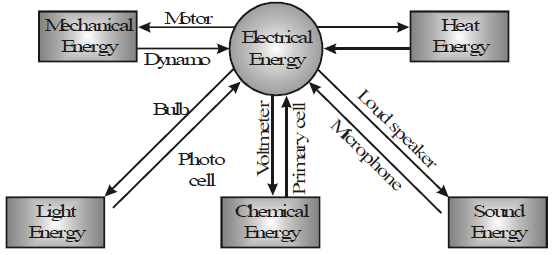Some man-made devices that convert one form of energy into another are is given as follows.

 Sr. Device Input energy Output energy 1. Fan Electrical energy Kinetic energy 2. Electric lamp Electrical energy Light energy 3. Electrical heaters Electrical energy Heat energy 4. Radio Electrical energy Sound energy 5. Water pump Electrical energy to kinetic energy of impellerto potential energy of water 6. Cell Chemical energy Electrical energy 7. Microphone Sound energy Electrical energy 8. Rechargeable cell (a) During discharging  Chemical energy(b) During charging Electrical energy (a) Electrical energy(b) Chemical energy 9. Loudspeaker Electrical energy Sound energy 10. Elevator moving up Electrical energy Potential energy 11. Television Electrical energy Sound energy, light energy 12. Thermal power plant Chemical energy of coal Electrical energy 13. Car Chemical energy of petrol/diesel Mechanical energy 14. Nuclear power plant Nuclear energy Electrical energy 15. Solar cell Solar energy Electrical energy 16. Watch Potential energy of wound spring K.E. of hands of watch 17. Generator Kinetic energy Electrical energy

## Power (Rate of Doing Work)

• Power of a machine is defined as the rate of work done by the machine.
• Power is defined as the rate of doing work.
• Power of a machine is defined as work done by the machine per second.
• Power is scalar quantity.

If you lift a block of mass 1 kg through a distance of 1 m in 2 seconds, what is the work done?

W = F x s = mg x h = 1 x 9.8 x 1 = 9.8 J

If you lift the same block through the same height in 1 minute, what is the work done?

The answer comes out to be the same 9.8 J.

What is the work done if the time taken is 5 minutes?

The work done is again 9.8 J.

But we are generally interested in time-oriented work, i.e., work should be completed in a particular amount of time. The physical quantity which takes care of 'how fast is the work done' is power.

Note: If we want to find rate of work done by a man then the word 'machine' can be replaced by 'man'.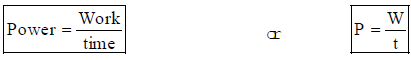### Unit of Power

SI unit of power is watt (W), 1 kW = 1000 W,

1 MW (Mega watt) = 106 W

Another unit of power is horse power (HP).

1GW(giga watt) = 109 W

1 HP = 746W

Definition of Watt

When t = 1 s, W = 1J, then P = 1 W

If the work done by a machine is 1 joule in a time of 1 second, then the power of the machine is 1 watt.

### Power in terms of Energy

Since work and energy are interconvertible, therefore,

Power = Energy/Time or

P = E/t

E = P x t

Also, W = F x s

When displacement is applied in the direction of force

then P = W/t  = F x s/t

⇒ P = F x v

This is power in terms of force and velocity.

### Average Power

Average power of an agent is defined as the ratio of total work done to the total time taken.

Average Power = (total work done /  total time taken)

## Kilowatt-hour (kWh)

One kilowatt-hour is the amount of energy consumed (or work done) by an agent in one hour working at a constant rate of one kilowatt.

### Is kWh a unit of power or energy?

We can write 1 kWh as 1 kW x 1h.

Now, since P = E/t

∴ E = P x t

If power is in kW (kilowatt) and time in hour, then the unit of energy is kWh.

The unit kWh is important because this is a commercial unit of energy used by electricity boards. If you enquire from your parents what was the last electricity bill? If the answer is 600 units, it means that you have used

600 kWh of energy during the duration of bill. Thus, you pay for the electrical energy that you use.

### Relation between kWh and Joule

1 kWh = 1000 Wh [as 1 kW = 1000 W]

Now, 1 W = 1 Js-1 and 1 h = 60 x 60 s = 3600 s

∴ 1kWh = 1000 Js-1 x 3600 s

= 3600000 J

= 3.6 x 106 J

= 3.6 MJ

∴ 1 kWh = 3.6 MJ

Note: An energy of 1 kWh is equivalent to using a bulb of 1 kW for 1 hour.

## Competitive Window

What can you conclude from the relationship,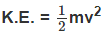we can conclude as follows :

When the velocity of a body is kept constant, the kinetic energy is directly proportional to the mass of the body, K.E. ∝ m

Thus,

• If the mass of a body is doubled (v remaining constant), the kinetic energy of the body also gets doubled.
• If the mass of the body is reduced to half (v remaining constant), the kinetic energy of the body also gets halved.

The kinetic energy of a body is directly proprotional to the square of its velocity (or speed) i.e., K.E. ∝ v2

So, (∵ m is constant)

• If the velocity of a body is doubled, then its kinetic energy increases four times.
• If the velocity of a body is reduced to half, then its kinetic energy gets to one-fourth.

How is the kinetic energy of a body related to its momentum

Let us consider a body of mass m having a velocity v. Then

Momentum of the body p = Mass x velocity = m x v

This gives, v = P/m .........(1)

From definition,

Kinetic energy (K.E.) of the body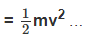... (2)

Substituting the value of v from Equation (1) into Equation (2) we can write,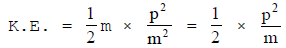Then, we can write, p2 = 2m x K.E.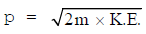Thus, Momentum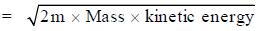The document Energy and Power | Science Class 9 is a part of the Class 9 Course Science Class 9.
All you need of Class 9 at this link: Class 9

## Science Class 9

66 videos|355 docs|97 tests

## FAQs on Energy and Power - Science Class 9

 1. Are various energy forms interconvertible?Ans. Yes, various energy forms are interconvertible. According to the law of conservation of energy, energy cannot be created or destroyed, but it can be converted from one form to another. For example, electrical energy can be converted into light energy in a light bulb, and chemical energy can be converted into thermal energy in a combustion reaction.
 2. What is power and how is it related to the rate of doing work?Ans. Power is the rate at which work is done or energy is transferred. It is the amount of work done or energy transferred per unit of time. Mathematically, power is calculated by dividing the work done or energy transferred by the time taken. Power is measured in watts (W) or kilowatts (kW).
 3. What is the commercial unit of energy?Ans. The commercial unit of energy is kilowatt-hour (kWh). It is commonly used to measure the amount of electrical energy consumed by households and businesses. One kilowatt-hour is equal to the amount of energy transferred or work done when a power of one kilowatt is applied for one hour.
 4. What is the competitive window for energy and power?Ans. The competitive window for energy and power refers to the period of time during which different energy sources or power generation technologies are compared and evaluated based on their efficiency, cost-effectiveness, and environmental impact. This window allows policymakers and investors to make informed decisions about the most suitable and sustainable energy options.
 5. What is the significance of studying energy and power in Class 9?Ans. Studying energy and power in Class 9 is significant as it provides a fundamental understanding of how energy works and its various forms. It helps in understanding the concept of work, power, and their units of measurement. This knowledge is essential for understanding the applications of energy in everyday life, as well as in various fields such as engineering, physics, and environmental science.

## Science Class 9

66 videos|355 docs|97 testsExplore Courses for Class 9 examSignup to see your scores go up within 7 days! Learn & Practice with 1000+ FREE Notes, Videos & Tests.
10M+ students study on EduRev
Track your progress, build streaks, highlight & save important lessons and more!
Related Searches

,

,

,

,

,

,

,

,

,

,

,

,

,

,

,

,

,

,

,

,

,

;